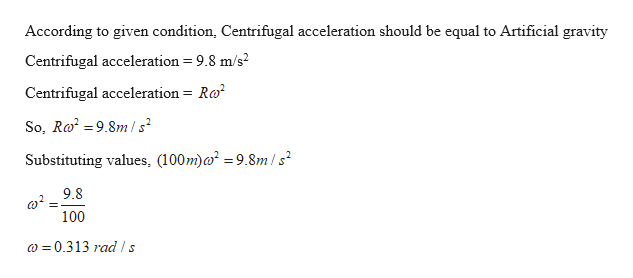A rotating space station is said to create "artificial gravity"-a loosely defined term used for an acceleration that would be crudely similar to gravity.  The outer wall of the rotating space station would become a floor for the astronauts, and the centripetal acceleration supplied by the floor would allow astronauts to exercise and maintain muscle and bone strength more naturally than in non-rotating space environments.  If the space station is 200 m in diameter, what angular velocity would produce an "artificial gravity" of 9.80 m/s2?Group of answer choices0.313 rad/s0.0980 rad/s10.2 rad/s3.19 rad/s

Question
A rotating space station is said to create "artificial gravity"-a loosely defined term used for an acceleration that would be crudely similar to gravity.  The outer wall of the rotating space station would become a floor for the astronauts, and the centripetal acceleration supplied by the floor would allow astronauts to exercise and maintain muscle and bone strength more naturally than in non-rotating space environments.  If the space station is 200 m in diameter, what angular velocity would produce an "artificial gravity" of 9.80 m/s2?
Group of answer choices
Step 1

Given:

Diameter of the space station,D = 200 m

Radius, R = 100 m

Artificial gravity(Acceleration) = 9.8 m/s2

Step 2

Calculating the value of angular velocity such that the value of artificial gravity is 9.8 m/s2:

When the station spins, centrifugal force acts...help_outlineImage TranscriptioncloseAccording to given condition, Centrifugal acceleration should be equal to Artificial gravity 9.8 m/s Centrifugal acceleration Centrifugal acceleration= Ra So, Ro2 =9.8m/s Substituting values, (100m)a2 = 9.8m/s2 9.8 100 0.313 rad ls fullscreen

Want to see the full answer?

See Solution

Want to see this answer and more?

Our solutions are written by experts, many with advanced degrees, and available 24/7

See Solution
Tagged in

Gravitation